# What Are Quantum Effects and How Do They Enable Quantum Information Science?

1. What’s so special about quantum information science?
2. What is quantum?
3. Quantum mechanics
4. Quantum physics
5. Quantum chemistry
6. Quantum computational chemistry
7. Quantum biology
8. Quantum field theory
9. Quantum theory
10. Quantum system
11. Isolated quantum system
12. Quantum effects
13. Quantum resource
14. Quantum state
15. Wave function
16. Linear algebra
17. Quantum information
18. Quantum bit — qubit
19. Stationary, flying, and shuttling qubits
20. Measurement / observation
21. Observable
22. Quantum phenomena
23. Macroscopic quantum phenomena
1. Discrete
2. Quanta
3. Particle and wave duality
4. Probabilistic
5. Uncertainty
6. Superposition
7. Schrödinger’s cat
8. Cat state
9. Entanglement
10. Spooky action at a distance
11. Bell’s theorem and Bell’s inequality
12. Bell states
13. Phase
14. Interference
15. Wave function
16. Density matrix or density operator
17. Probability amplitude
18. Unitarity principle
19. Basis state
20. Computational basis state
21. Quantum state
22. State vector
23. Collapse of wave function on measurement
24. Measurement
25. No-cloning theorem
26. Hamiltonian
27. Schrödinger’s equation
28. Time evolution
29. Fermi-Dirac statistics
30. Bose-Einstein statistics
31. Fermions
32. Bosons
33. Pauli exclusion principle
34. Spin
35. Integer spin
36. Half-spin or half-integer spin or spin 1/2
37. Spin up and spin down
38. Cooper pairs
39. Superconductivity and superfluidity
40. Tunneling
41. Josephson effect
42. Quantum hall effect
43. Macroscopic quantum effects
44. Zero-point energy and vacuum fluctuations
1. Quantum computing offers much greater performance than classical computing through quantum parallelism which offers an exponential speedup — evaluating many (all) possibilities in parallel, in a single calculation.
2. Quantum communication offers inherent security through quantum entanglement — also known as spooky action at a distance, in contrast to security as a problematic afterthought for classical communication and networking.
3. Quantum metrology and quantum sensing offer much greater accuracy and precision for measurements of physical quantities and detection of objects.
1. Discrete rather than continuous values for physical quantities.
2. Quanta for discrete values. The unit for discrete values. Technically, quantum is a singular unit and quanta is the plural of quantum (just as with data and datum.)
3. Particle and wave duality. Particles have wave properties and behavior, and waves have particle properties and behavior. For example, a photon can act as a particle as well as a wave, and an electron can act as a wave as well as a particle.
4. Probabilistic rather than strictly deterministic behavior.
5. Uncertainty of exact value or measurement. More than just uncertainty of any measurement, there is uncertainty in the actual value of any property, as a fundamental principle of quantum mechanics. A given property of a given quantum system may have a range of values, even before the property is measured. For example, a particle or wave can be at two — or more — positions at the same moment of time.
6. Superposition of states — a quantum system can be in two quantum states at the same time. See spin up and spin down.
7. Schrödinger’s cat — a thought experiment which demonstrates superposition — the cat can be both alive and dead at the same time.
8. Cat state — alternate name for superposition of two quantum states. A tribute to Schrödinger’s cat.
9. Entanglement — the same quantum state can exist at two physically separated locations at the same time.
10. Spooky action at a distance — popular reference to entanglement.
11. Bell’s theorem and Bell’s inequality — quantum mechanics cannot be explained simply by adding “hidden variables” to classical mechanics.
12. Bell states — the various combinations of quantum states for two entangled particles. The quantum states of the two particles are correlated — they may be identical quantum states, but they don’t have to be identical. Measuring the state of one particle will allow the observer to infer the state of the other particle. This is useful for both quantum computing and quantum communication.
13. Phase — the complex or imaginary part of the probability amplitude of a quantum state. The notion of cyclical or periodic behavior or a fraction of a single cycle of a wave or circle. Measured either in radians (two pi radians in a circle or cycle) or a fraction between 0.0 and 1.0, where 1.0 corresponds to a full, single cycle or circle (two pi radians.)
14. Interference — cancellation or reinforcement of the complex or imaginary part of the probability amplitude of two quantum states (phases). Useful for quantum computing — it enables quantum parallelism. Not to be confused with environmental interference which disrupts the operation of a quantum system.
15. Wave function is used to fully describe the state of a particle or wave (technically, an isolated quantum system) based on the probabilities of superposed and entangled states. The sum of the basis states of the quantum system, each weighted by its probability amplitude. Linear algebra is the notation used to express a wave function.
16. Density matrix or density operator — an alternative to the wave function for describing the quantum state of a quantum system.
17. Probability amplitude — a complex number with both real and imaginary parts which represents the likelihood of the quantum system being in a particular state, a basis state. Square it and then take the square root to get the probability for a particular basis state. The imaginary part of the probability amplitude is also referred to as its phase.
18. Unitarity principle — the probabilities (square root of the square of the probability amplitude) of all possible states of a quantum system must sum to 1.0 by definition.
19. Basis state — the actual numeric value of a single quantum state, comparable to a binary 0 or 1.
20. Computational basis state — the combined basis states of a collection of qubits. A collection of strings of 0’s and 1’s, each string having a probability amplitude as its weight in the wave function. Essentially each string is an n-bit binary value.
21. Quantum state — the state of an isolated quantum system described by its wave function. Alternatively, a single basis state.
22. State vector — a single-column matrix describing the state of a quantum system, where each row represents a computational basis state and the value in the row represents the probability amplitude for that computational basis state. For a single qubit there would be two rows, for two qubits there would be four rows, and for k qubits there would be 2^k rows. Commonly referred to as a ket, the right hand side of bra-ket notation.
23. Collapse of wave function on measurement — the probabilities of superposed states will influence but not completely determine the observed value. Measurement always causes the wave function of a quantum system to collapse — permanently.
24. Measurement — the process of observing a quantum system. By definition, measurement causes collapse of the wave function, and will always produce a single basis state (0 or 1) or computational basis state (string of 0’s and 1’s) regardless of any superposition or entanglement which may be defined by the wave function of the quantum system.
25. No-cloning theorem — quantum information (quantum state) cannot be copied — attempting to read (measure or observe) quantum state causes it (its wave function) to collapse to a discrete, non-quantum state.
26. Hamiltonian — an equation which expresses the energy of a quantum system.
27. Schrödinger’s equation — describes the evolution of the quantum state of a quantum system over time in terms of its Hamiltonian and wave function.
28. Time evolution — how the quantum state of a quantum system incrementally evolves over time based on its Hamiltonian and wave function. See Schrödinger’s equation.
29. Fermi-Dirac statistics — rules for fermions which require them to obey or follow the Pauli exclusion principle.
30. Bose-Einstein statistics — rules for bosons which allow them to violate (not follow) the Pauli exclusion principle. Sometimes referred to as BES.
31. Fermions — elementary particles, such as electrons, and atoms which obey Fermi-Dirac statistics, including the Pauli exclusion principle. Fermions have half-spin (half-integer spin or spin 1/2) by definition. Fermions can collide, bounce apart, or form composite particles, even molecules.
32. Bosons — elementary particles, such as photons, and some atoms under special circumstances, which obey or follow Bose-Einstein statistics, which permits them to violate (not follow) the Pauli exclusion principle. Bosons have integer-spin by definition. Bosons can pass right through each other, or even occupy the same position, without interacting.
33. Pauli exclusion principle — no two fermions, particles with half-spin, such as electrons and most atoms, can occupy the same quantum state (e.g., position) at the same time. In contrast, bosons, such as photons and pairs of fermions in special circumstances, can occupy the same quantum state (e.g., position) at the same time.
34. Spin — the angular momentum, much like a spinning gyroscope, of an elementary particle or an atom.
35. Integer spin — the form of angular momentum of bosons which allows them to violate the Pauli exclusion principle. Bosons obey or follow Bose-Einstein statistics, which allow them to occupy the same quantum state (e.g., position.)
36. Half-spin or half-integer spin or spin 1/2 — the form of angular momentum of fermions which requires them to obey or follow the Pauli exclusion principle, preventing them from occupying the same quantum state (e.g., position.)
37. Spin up and spin downspin of a particle can have a direction of spin, which is the direction of the vector representing the angular momentum, and up and down are the direction of the axis of rotation, based on using the right-hand rule, where curled fingers represent the direction of spin and the pointing thumb represents the axis of rotation, pointing either up or down as the thumb points. Spin up and spin down can be used to represent two basis states for both quantum computing and quantum communication. Spin up and down for a particle can in fact be superposed, so that a particle (e.g., qubit) is in a linear combination of both spin up and spin down, corresponding to a linear combination of a 0 and a 1.
38. Cooper pairs — electrons can pair up at ultracold temperatures (near absolute zero), so that the pair acts as a single boson rather than as a pair of fermions since the half-spin of each of the two fermions add up to be the integer spin of a boson. This allows the pair to violate the Pauli exclusion principle, enabling superconductivity.
39. Superconductivity and superfluidity — the phenomenon of zero resistance, for electrical conduction and mechanical flow respectively, which occurs at ultracold temperatures (near absolute zero), allowing pairs of fermions, such as electrons and helium atoms, to pair up, which transforms the half-spin of each fermion into integral spin for the pair, allowing the pair to act as a boson, which no longer must obey the Pauli exclusion principle, so that the pairs can now occupy the same position, which is permitted for bosons which obey Bose-Einstein statistics, but is not possible for fermions alone, which must obey Fermi-Dirac statistics.
40. Tunneling — the ability of a subatomic particle or wave such as an electron to appear to be able to move through a solid barrier as if it weren’t there. In actuality, quantum mechanics dictates that a particle or wave has a probability to be at any given location, so that a particle or wave can have a probability of being at either side of the barrier at a given moment, allowing the particle or wave to appear to skip over or through the barrier in the next moment. An example would be electrons and a Josephson junction used in a superconducting transmon qubit.
41. Josephson effecttunneling of Cooper pairs of electrons through an insulating barrier. Used to implement Josephson junctions, such as for superconducting transmon qubits. Also has applications for quantum sensing and quantum measurement.
42. Quantum hall effect — quantized conductance (flow of current or electrons) induced by a magnetic field at very low temperatures. Useful for precise measurements.
43. Macroscopic quantum effects — quantum effects which can be observed at the macroscopic scale — above the level of the atomic, subatomic, and molecular scale, such as superconductivity, superfluidity, and the quantum hall effect.
44. Zero-point energy and vacuum fluctuations — uncertainty means that even a vacuum is not at absolutely zero energy. A vacuum fluctuates within the range of minimum uncertainty. Although an application to quantum information science is not clear at present, specialized hardware can be used to allow a classical computer to measure the fluctuating zero-point energy of a vacuum to generate true random numbers — which cannot be calculated by a strict Turing machine alone.
45. Creation and annihilation operators — primarily to facilitate quantum mechanical modeling of many-particle systems.
1. The quantum state is described by a wave function.
2. The individual possible states are known as basis states. Such as a 0 and a 1.
3. Each basis state in a wave function occurs with some probability.
4. A basis state can also have a complex or imaginary component, known as a phase, which is periodic or cyclical. This is exploited in quantum computing to enable quantum parallelism using interference of the phase of a potentially large number of quantum states.
5. The probability and phase are combined into a single, complex value, called the probability amplitude, where the probability is the square root of the absolute value (or modulus) of the complex number.
6. The basis states and their probability amplitudes are combined to form the wave function.
7. If the probability of a basis state is other than 0.0 or 1.0, the two basis states are superposed.
8. The quantum states of two separate particles or waves — two isolated quantum systems — can be shared or entangled.

1. The quantum hypothesis — in general. Historical role in transitioning from the classical world to the quantum world.
2. Momentum, angular momentum, orbital momentum.
3. Pure state, mixed state, density matrix, density operator.
4. Wave packets.
5. Nuclear magnetic resonance (NMR). Some early efforts in quantum computing relied on NMR.
6. Nuclear electric resonance.
7. Gravity. Nominally lies in the realm of General Relativity rather than quantum mechanics, but… who knows for sure. Can a quantum computer be used to accurately simulate or model gravity and gravitational effects, including effects on time and space?

# Alternative titles

1. Quantum effects
2. Quantum effects for quantum computing
3. Quantum effects for quantum information science
4. What are quantum effects?
5. Superficial introduction to quantum effects for non-physicists
6. What are quantum effects and how do they relate to quantum computing?
7. What are quantum effects and how do they relate to quantum information science?
8. Quantum effects — The magic sauce which enables quantum information science
9. Quantum effects — The magic sauce which enables quantum computing
10. What are Quantum effects and how do they enable quantum information science?

--

--

--

## More from Jack Krupansky

Freelance Consultant

Love podcasts or audiobooks? Learn on the go with our new app.

## Recommended from Medium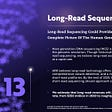## A three-dimensional solar cell## Fields Medalist Martin Hairer Sits Down With PIMS## Do whales get cancer more often than mice?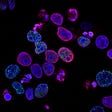## Peter Shor — pioneer of quantum computing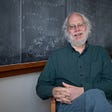## Quantum Computing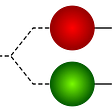## The floating mountains of Pandora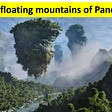## The fall of the science giants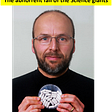## Jack Krupansky

Freelance Consultant

## Podcast with James Sanders, 451 Research## What I Learned From Fire Opal…## “Is a quantum computer some sort of cake?”## An Introduction to Quantum Computing For African Youth